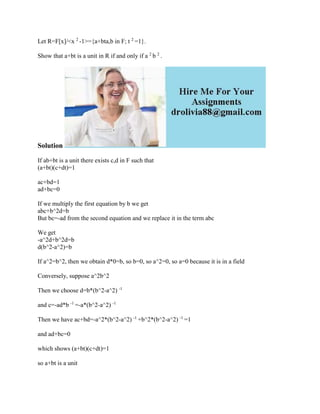Seu SlideShare está sendo baixado. ×

# Let R-F-x---x2-1--{a+bta-b in F- t2-1}- Show that a+bt is a unit in R.docx

Anúncio
Anúncio
Anúncio
Anúncio
Anúncio
Anúncio
Anúncio
Anúncio
Anúncio
Anúncio
AnúncioCarregando em…3
×

1 de 2 Anúncio

# Let R-F-x---x2-1--{a+bta-b in F- t2-1}- Show that a+bt is a unit in R.docx

Let R=F[x]/<x 2 -1>={a+bta,b in F; t 2 =1}.
Show that a+bt is a unit in R if and only if a 2 b 2 .
Solution
If ab+bt is a unit there exists c,d in F such that
(a+bt)(c+dt)=1

ac+bd=1

If we multiply the first equation by b we get
abc+b^2d=b
But bc=-ad from the second equation and we replace it in the term abc

We get
-a^2d+b^2d=b
d(b^2-a^2)=b

If a^2=b^2, then we obtain d*0=b, so b=0, so a^2=0, so a=0 because it is in a field

Conversely, suppose a^2b^2
Then we choose d=b*(b^2-a^2) -1
Then we have ac+bd=-a^2*(b^2-a^2) -1 +b^2*(b^2-a^2) -1 =1
which shows (a+bt)(c+dt)=1
so a+bt is a unit
.

Let R=F[x]/<x 2 -1>={a+bta,b in F; t 2 =1}.
Show that a+bt is a unit in R if and only if a 2 b 2 .
Solution
If ab+bt is a unit there exists c,d in F such that
(a+bt)(c+dt)=1

ac+bd=1

If we multiply the first equation by b we get
abc+b^2d=b
But bc=-ad from the second equation and we replace it in the term abc

We get
-a^2d+b^2d=b
d(b^2-a^2)=b

If a^2=b^2, then we obtain d*0=b, so b=0, so a^2=0, so a=0 because it is in a field

Conversely, suppose a^2b^2
Then we choose d=b*(b^2-a^2) -1